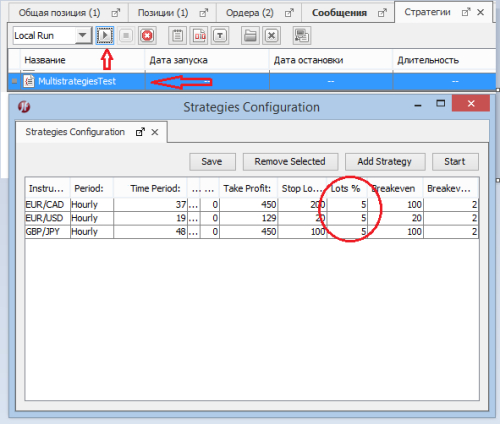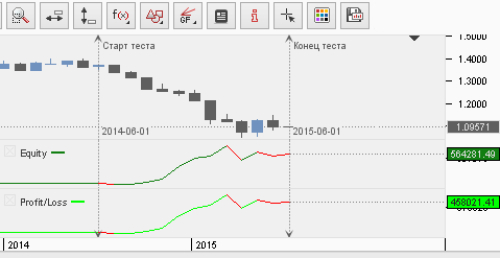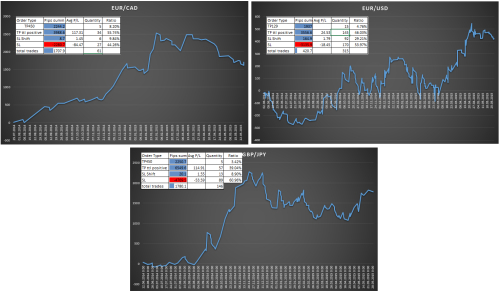So, how to manage within account, when you have a few strategies, that have a different profit and loss?
Let’s take a look on a several variants, that've been tested on a historical tester on Jforex platform:
1. Multiple Strategies. This method of managing, when you have one account and one or more strategies that trades the percentage of the deposit. The formula for calculating the lot size for trading percentage in my case, is calculated as
amount=((((equity*leverage)/100)*lots)/1000000) .

 [i]Example: amount= ((((150 000 * 100)/100)* 5)/1 000 000 = 0.75 lot.
(The formula does not claim to originality, I had seen either in Dukas Wiki, or in the forum of Dukascopy. The reasons for the revision of it, did not arise.)
[/i]

According to this formula, volumes of trades will be calculated throughout the article.
To check the profitability of strategies I used Multiple_strategies. We connect our strategy to it. Start a strategy and set a parameters.I set a percentage for all strategies, giving a total of 15% of the total capital by the formula that is written above.
If you count the risks by formula:
 [i]SL * Lots / 100”
you get 16% of the risk for each position, as follows:
• EUR/CAD risk = 200 * 5 / 100 = 10% - risk per order.
• EUR/USD risk = 20 * 5 / 100 = 1 % - risk per order.
• GBP/JPY risk = 100 * 5/ 100 = 5 % - risk per order.
 Summ of risks = 10%+1%+5% = 16%, avaregae risk = (10+1+5)/3 = 5.333%

The risks are set randomly. But further, you can set the level of risk for each strategy separately, considering their PL factor (the ratio of profit and loss), and based on your own system of money management.
With specified parameters, we launch our strategy and test it in the interval from 2014-06-01 00:00:00 to 2015-06-01 00:00:00. We get the result:Result of test on 1 month time frame.As we see, the profit indeed is, and if we look at the monthly chart we can see stability. With starting conditions in \$ 150,000 we received the final deposit of \$ 564280.41, which amounts in a profit of 376.2%, and the commission amounted to \$ 43779.98
But we are now wondering how much each strategy can bring individually. To do this, we will open a table results in excel. Add up number of pips as seen in the picture below of range from the data (Profit/Loss in pips) and to build diagram.And so we have the following results: EUR/CAD - 1707.9 pips, EUR/USD - 420.7, GBP/JPY - 1780.1
Profit chart in pips and other results are as follows:The plate specifies analysis of orders.
Instruction based on example of EUR/CAD:
TP450 - all positions closed by TP of 450 pips; Pips summ - the total amount of pips; Avg P / L - The average value of the order; Quantity - quantity of orders; Ratio - ratio of orders to the total number of orders; Tp ttl positive - all positive orders closed by Take Profit and by strategy signals; SL Shift- orders are closed in plus by moved Breakeven; SL- any negative orders closed by stop loss and signals strategy.

As we see all the results are profitable. But our task is to find the most effective method of account management. So now, I present to you the results of trade, each trading strategy in a separate account

2. One Account - One Strategy. In the first method, the conditions have been so - Account - \$ 150,000 and the use of volume - by 5% for each strategy. Now we will have three accounts by \$ 50,000 and to keep the proportion of capital management, we will use 15% to trade on each deposit.The amount of the final deposit for each account amounted to \$ 352444.60 (+ 235%). The Commission - \$ 35650.34.
I confess to you, for me results were a complete surprise. Because I believed that the loss to one account will not affect the other, and the profit will be more, but in fact it turned out, 200k lost profits, despite the fact that the total commission in the second case is only 35650.34 (the reason why this happened, read in the output)
Let's see, maybe the third method shows the best results.

3. Fixed amount. As in the second case, we create three accounts of \$ 50,000. We take 15% of every deposit that will be at 0.75 lot for each order, for each currency pair, and we will trade this amount fixed for a year. Regardless of changes in equity, the amount will be 0.75 lots for each order. We get the results of trade:And so the profit was \$ 396,626.74 (+ 264.4%), with net commission \$ 19,299.91. Moreover profit chart looks more optimistic than in example of One Account - One Strategy. Advantages of this method is that if the strategy shows poor results for a long time, but in the sum gives a positive result, it is better to say on the account, and their loss easier to go through psychologically, than at percentage rate of the deposit, like in the second case .Picture #1 - One Account - One Strategy. Picture #2 - Fixed amount.

And as you may have noticed that a series of consecutive losses have a negative effect on the account, where the volume of order is a percentage of equity.

Conclusions: